Courses

# Solutions of Magnetic Effects of Electric Current (Page No- 74) - Physics By Lakhmir Singh, Class 10 Class 10 Notes | EduRev

## Class 10 : Solutions of Magnetic Effects of Electric Current (Page No- 74) - Physics By Lakhmir Singh, Class 10 Class 10 Notes | EduRev

The document Solutions of Magnetic Effects of Electric Current (Page No- 74) - Physics By Lakhmir Singh, Class 10 Class 10 Notes | EduRev is a part of Class 10 category.
All you need of Class 10 at this link: Class 10

Lakhmir Singh Biology Class 10 Solutions Page No:74

Question 24: Copy the figure given below which shows a plotting compass and a magnet. Label the N pole of the magnet and draw the field line on which the compass lies.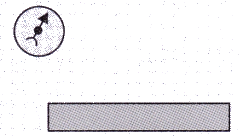Solution : As the north pole of the magnetic needle is pointing in the opposite direction,so the nearer end of the magnet will be north pole.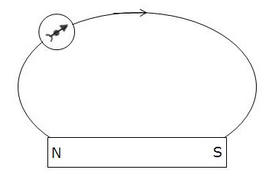Question 25: (a) The diagram shows a bar magnet surrounded by four plotting compasses. Copy the diagram and mark in it the direction of the compass needle for each of the cases B, C and D.
(b) Which is the north pole, X or Y ?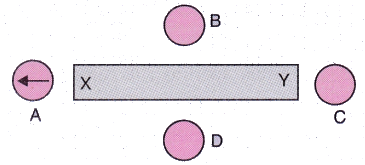Solution :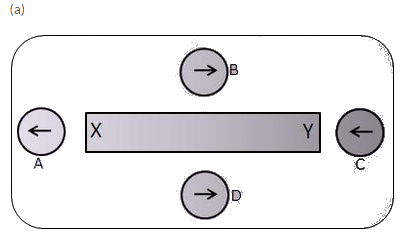(b) X, as it repels the north pole (tip) of magnetic needle.

Question 26: The three diagrams in the following figure show the lines of force (field lines) between the poles of two magnets. Identify the poles A, B, C, D, E and F.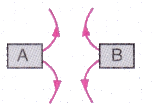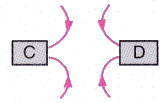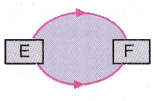Solution : A = N; B = N; C = S; D = S; E = N; F = S

Question 27: The figure given below shows the magnetic field between two magnets :
(i) Copy the diagram and label the other poles of the magnets.
(ii) Which is the weaker magnet ?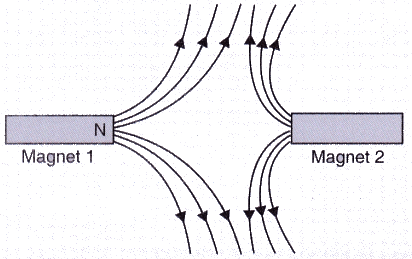Solution :
(a)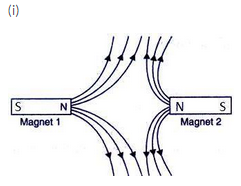(ii) Magnet 2 is weaker.

Offer running on EduRev: Apply code STAYHOME200 to get INR 200 off on our premium plan EduRev Infinity!

,

,

,

,

,

,

,

,

,

,

,

,

,

,

,

,

,

,

,

,

,

,

,

,

;